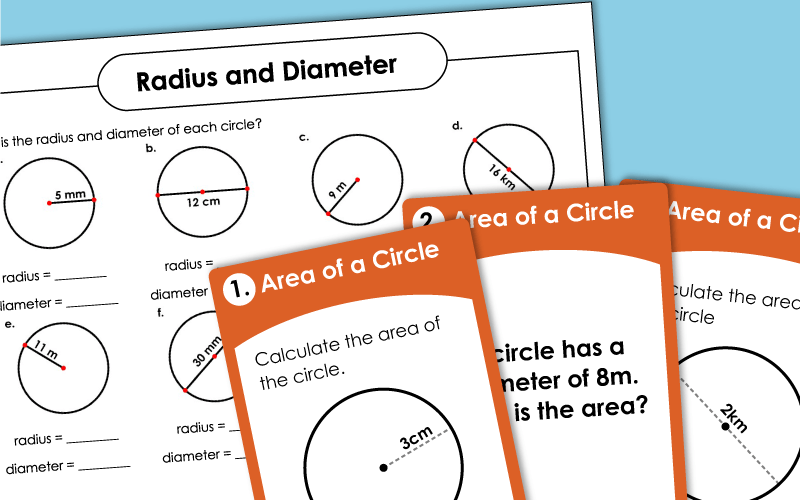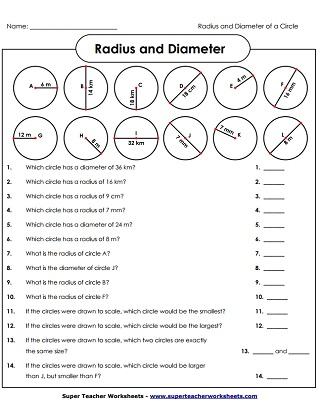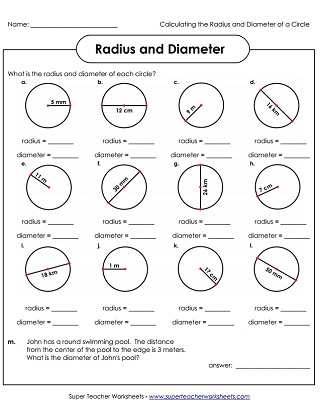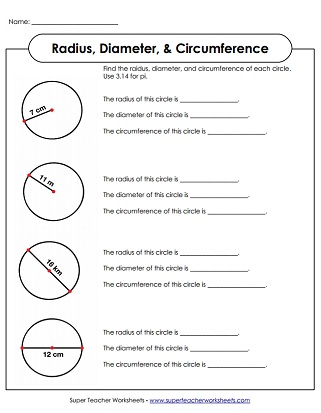# Circles: Radius, Diameter, Circumference, Area

Print out these worksheets on circles.  Practice naming circles and calculating circumference, radius, diameter, and area of a circle.## Circumference and Area (Intermediate)

The diameter of each circle is shown.  Students must calculate the circumference, or the distance around the circle.
The radius of each circle is drawn. Pupils must figure out the distance around the edge of the circle, or circumference.
Each circle picture shows a radius or diameter.  Students must use this information to figure out the circumference.
Students measure various circular objects and divide the circumference by the diameter to get pi.
A radius is drawn on each circle shape.  Find the area of the circle.
Either the diameter or the radius is shown on each shape. Calculate the area for each.
Use the formula pi-r-squared to calculate the area of each circle.
Determine the area of each circle. Includes a sample problem at the top.
When your students sing the pi song, they'll never forget how to calculate the circumference and area of a circle.
Each task card has an area problem for students to solve. Use these for peer practice sessions, small group instruction, or on your document camera.

Can you name the radius and diameter of each of these circles?
Find the diameter and radius of  each circle.  Also, figure out which circles are the largest, which are the smallest, and which are the same size.
Determine the diameter, radius, and circumference of each of these circles.

## Also on Super Teacher Worksheets...

Geometry Worksheets

Worksheets on angles, perimeter, area, lines, polygons, and more!

Symmetry Worksheets

Find lines of symmetry, identify symmetrical shapes, and sketch symmetrical figures.

Brain Teaser Worksheets

Can your students solve these brain bafflers?

## Worksheet ImagesMy Account
Site Information